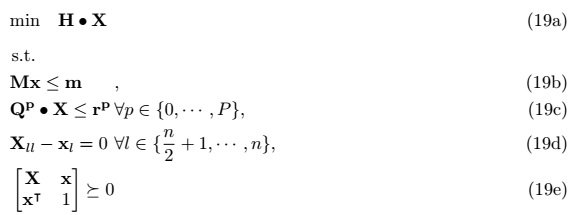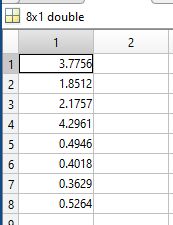# Infeasible Status for SDP Program

I have an SDP program which is a relaxation of non-convex quadratically constrained quadratic program. Here is my SDP program formulation:Where n is my number of decision variables (n = 8 in this case), 19b is the LMI for all the linear inequalities, 19c are all the quadratic inequalities (note that there are multiple data matrices Q), 19d to enforce the binary decision variables from n/2 + 1 to n (the second half of the vector x) and 19e to enforce semidefinitness over X (X = xx^T)

Attached the full program with the all the required data matrices:
FULL MATLAB SCRIPT

Here is the output I got from CVX:

``````Calling SDPT3 4.0: 73 variables, 33 equality constraints
------------------------------------------------------------

num. of constraints = 33
dim. of sdp    var  =  9,   num. of sdp  blk  =  1
dim. of linear var  = 28
*******************************************************************
SDPT3: Infeasible path-following algorithms
*******************************************************************
version  predcorr  gam  expon  scale_data
HKM      1      0.000   1        0
it pstep dstep pinfeas dinfeas  gap      prim-obj      dual-obj    cputime
-------------------------------------------------------------------
0|0.000|0.000|7.5e+01|9.9e+00|4.4e+04|-8.082054e+02  0.000000e+00| 0:0:00| chol  1  1
1|0.652|0.844|2.6e+01|1.8e+00|1.5e+04|-6.101845e+02  1.869678e+02| 0:0:00| chol  1  1
2|0.575|0.962|1.1e+01|1.5e-01|7.5e+03|-2.091287e+02  1.032368e+03| 0:0:00| chol  1  1
3|0.167|1.000|9.2e+00|2.5e-02|1.1e+04|-1.798682e+02  2.276281e+04| 0:0:00| chol  1  1
4|0.079|1.000|8.5e+00|7.6e-03|4.9e+04|-1.633760e+02  2.781323e+06| 0:0:00| chol  1  1
5|0.011|1.000|8.4e+00|2.3e-03|2.5e+07|-1.607972e+02  1.903454e+10| 0:0:00| chol  1  1
6|0.011|0.011|8.3e+00|2.3e-03|2.5e+12|-8.344242e+01  1.174126e+14| 0:0:00| chol  1  2
7|0.000|0.000|8.3e+00|2.3e-03|2.5e+12|-2.104616e+02  1.175206e+14| 0:0:00| chol  1  2
8|0.000|0.000|8.3e+00|2.3e-03|2.6e+12|-1.354355e+02  1.180461e+14| 0:0:00| chol  1  2
9|0.000|0.000|8.3e+00|2.4e-03|2.6e+12|-1.403248e+02  1.189495e+14| 0:0:00| chol  1  2
10|0.000|0.000|8.3e+00|2.4e-03|2.6e+12|-1.475756e+02  1.199959e+14| 0:0:00| chol  1  2
11|0.000|0.000|8.3e+00|2.3e-03|2.6e+12|-1.461515e+02  1.211484e+14| 0:0:00| chol  1  2
12|0.000|0.000|8.3e+00|2.3e-03|2.9e+12|-1.447358e+02  1.345997e+14| 0:0:00| chol  1  2
13|0.000|0.000|8.3e+00|2.3e-03|3.9e+12|-1.456245e+02  1.792255e+14| 0:0:00| chol  1  2
14|0.000|0.000|8.3e+00|2.3e-03|5.8e+12|-1.451428e+02  2.708846e+14| 0:0:00| chol  1  2
15|0.000|0.002|8.3e+00|3.5e-03|3.2e+13|-1.452762e+02  1.524154e+15| 0:0:00| chol  1  2
16|0.001|0.000|8.3e+00|3.5e-03|3.9e+13|-1.450579e+02  1.938151e+15| 0:0:00| chol  1  2
17|0.001|0.007|8.3e+00|2.0e-02|7.0e+14|-1.450150e+02  3.587140e+16| 0:0:00| chol  2  3
18|0.001|0.000|8.3e+00|3.9e-02|9.8e+14|-1.447792e+02  5.329337e+16| 0:0:00| chol  2  3
19|0.001|0.036|8.3e+00|4.8e+00|1.1e+17|-1.447188e+02  6.000215e+18| 0:0:00|
sqlp stop: primal problem is suspected of being infeasible
-------------------------------------------------------------------
number of iterations   = 19
residual of primal infeasibility
certificate (y,Z)      = 5.40e-18
reldist to infeas.    <= 4.65e-18
Total CPU time (secs)  = 0.10
CPU time per iteration = 0.01
termination code       =  1
DIMACS: 1.6e+01  0.0e+00  5.2e+00  0.0e+00  -1.0e+00  1.8e-02
-------------------------------------------------------------------

------------------------------------------------------------
Status: Infeasible
Optimal value (cvx_optval): +Inf
``````

If you have any questions, please let me know in the comments.

Except for section 1, follow the advice at https://yalmip.github.io/debugginginfeasible, which also applies to CVX.

Thank you sir for your comment!

I managed to get it work and the status now is solved! the problem was in my data matrices

I have an issue with the binary decision variables … the output result are not binary … do you have any suggestions ?The second half of this vector must be binary (0 or 1)

Your program doesn’t have any declared binary variables, nor is SDPT3 capable of handling them.

19d is not a binary constraint. It is an equality constraint on some variables. Nothing constrains those variables to be binary (zero or one).

1 Like

Actually in the code there is a linear constraint combined in the LMI 19b that enforces some of the decision variables to 0 <= x <= 1 then by the constraint 19d, I’m enforcing it to 0 or 1 for example:
x^2 - x = 0 (since X_ll is the diagonal of the matrix X which contains the decision variables to the power of 2) this constraint will be valid only when x is either 1 or zero.
Check equation (9) HERE it has a similar example for a binary decision variables {-1, 1}

Show us which (explicit) constraint in your program is violated (by more than solver tolerance).

Perhaps you are assuming the solution is rank one (X = x*x’ at optimality)? I don’t believe there is any such guarantee in this case.

In the attached program line 37 you will find this constraint:

``````(diag(X) .* Mult) - (x .* Mult) == 0;
``````

Where ` Mult = [zeros(4, 1) ;ones(4, 1)];` and is used to enforce constraint 19d. The reason why I multiply by `Mult` that is because the second half of the decision variables’ vector are binary variables.
Nope I’m not assuming the solution is rank one. Constraint 19e is used only to enforce `X = x * x'` and since this constraint is non-convex, I relaxed it to `X >= xx'` which is implied by the Schur’s complement block matrix in constraint 19e.

1 Like

My answer is the same. You have relaxed the original constraint. Assuming the original constraint holds with equality is the same as assuming a rank one solution. That is the fallacy of your approach. You have not discovered a magic convex formulation to solve a non-convex problem. You are getting s smaller (better) objective value than the unrelaxed problem because the optimization is 'taking advantage of" the relaxation.

None of your explicit constraints are being violated. Only the fallaciously implied constraints in your head, which do not in fact follow from the explicit constraints.

1 Like

So the only way to enforce the binary constraint on some of the decision variables is by using heuristics (approximations of the solution), am I right ?

1 Like

You could declare binary variables. Unfortunately, there currently is no solver available under CVX which can solve Mixed-Inetger Semidefinite programs, unless CVX is able to convert the semidefinite constraints to SOCP constraints. which it won’t be able to do in your case because the length of x > 2.

YALMIP will let you (attempt to) solve such a problem using YALMIP’s BNB as solver, using a linear SDP solver, such as SDPT3, SeDuMi, or Mosek called by BNB to solve continuous SDP subproblems…

1 Like